Definitions of Square Dance Calls and Concepts
Initially Concept [C3A]

Index -->  Plus  |  A1  |  A2  |  C1  |  C2  |  C3A  |  C3B  |  C4  |  NOL  |
Definitions (Text Only) -->  Plus  |  A1  |  A2  |  C1  |  C2  |  C3A  |  C3B  |  C4  |  NOL  |
 Find call:

 \$B8@8l(BInitially anyConcept anything

Do the first part of the anything call using the given CONCEPT; then do the remainder of the anything call (i.e., without using the given CONCEPT). EN: 10
anything \$B\$N%3!<%k\$N;O\$a\$N%Q!<%H\$r(B, \$BM?\$(\$i\$l\$?(B CONCEPT \$B\$G9T\$\$\$^\$9!%\$=\$7\$F(B, anything \$B\$N%3!<%k\$N;D\$j\$r9T\$\$\$^\$9(B (\$B\$9\$J\$o\$A(B, \$BM?\$(\$i\$l\$?(B CONCEPT \$B\$r;H\$o\$:\$K(B)\$B!%(B JP: 10

\$BCm
• The anything call must have a well-defined first partEN: 20
anything \$B\$N%3!<%k\$K\$O(B, first part \$B\$,L@3N\$KDj5A\$5\$l\$F\$\$\$kI,MW\$,\$"\$j\$^\$9!%(B update JP: 20
• After doing the first part of the anything call using the given CONCEPT, re-evaluate your position within the resulting formation and apply the definition of the remainder of the call. EN: 30
anything \$B\$N%3!<%k\$N;O\$a\$N%Q!<%H\$r(B, \$BM?\$(\$i\$l\$?(B CONCEPT \$B\$G9T\$C\$?8e(B, \$B7k2L\$N(B formation \$B\$K\$*\$1\$k<+J,\$N0LCV\$r:FI>2A(B (re-evaluate) \$B\$7(B, \$B;D\$j\$N%3!<%k\$rDj5ADL\$j9T\$\$\$^\$9!%(B JP: 30
• Initially is a meta-concept (a concept that requires another concept as an argument). The caller can not say Initially anything. The caller must give a concept after the word InitiallyEN: 40
Initially \$B\$O(B meta-concept (\$B%a%?%3%s%;%W%H(B, \$BJL\$N(B concept \$B\$r0z?t\$H\$7\$FMW5a\$9\$k(B concept) \$B\$G\$9!%%3!<%i!<\$O(B Initially anything \$B\$H\$O8@\$(\$^\$;\$s!%(BInitially \$B\$N8@MU\$N8e\$KI,\$:(B concept \$B\$r8@\$o\$J\$1\$l\$P\$J\$j\$^\$;\$s!%(B
\$BLuJP: 40

•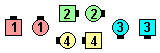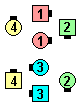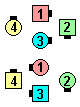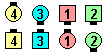\$B\$NA0(B Siamese Diamond Circulate\$B\$N8e(B Very Centers Trade\$B\$N8e(B Centers Cast Off 3/4\$B\$N8e(B (\$B=*\$o\$j(B)
Initially Twice Triple Star Thru :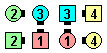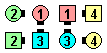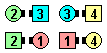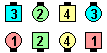\$B\$NA0(B (Centers)Star Thru Twice EN: 50 AddJapanese \$B\$N8e(B (Centers)Mirror Star Thru\$B\$N8e(B Star Thru\$B\$N8e(B (\$B=*\$o\$j(B)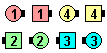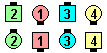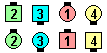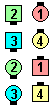\$B\$NA0(B Tandem Hinge\$B\$N8e(B Centers Trade\$B\$N8e(B Step & Fold\$B\$N8e(B (\$B=*\$o\$j(B)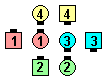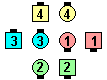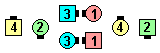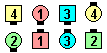\$B\$NA0(B As Couples Scoot Back\$B\$N8e(B Centers Cast Off 3/4 as Outsides Cast Back & Touch 1/2\$B\$N8e(B Centers Counter Rotate 1/4 as Outsides Step & Fold\$B\$N8e(B (\$B=*\$o\$j(B)
Initially Eight By Recoil: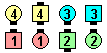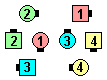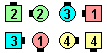\$B\$NA0(B Eight By Box Recycle\$B\$N8e(B Step & Fold\$B\$N8e(B (\$B=*\$o\$j(B)
Initially Checker Box Remake: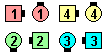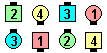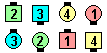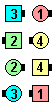\$B\$NA0(B Checker Box Right Arm Turn 1/4\$B\$N8e(B Centers Left Arm Turn 1/2\$B\$N8e(B Right Arm Turn 3/4\$B\$N8e(B (\$B=*\$o\$j(B)

(starting from Trade By formation): EN: 60
Trade By formation \$B\$+\$i(B, \$BJP: 60
• Initially Stretched Box Sidetrack:

Centers Pass Thru & all Zig-Zag; all 8 Counter Rotate 1/4; Roll. Ends in Double Pass Thru. EN: 70
Centers Pass Thru & all Zig-Zag, all 8 Counter Rotate 1/4, Roll. Double Pass Thru \$B\$G=*\$o\$j\$^\$9!%(B JP: 70
• Stretched Box Sidetrack:

Centers Pass Thru & all Zig-Zag; Split Counter Rotate 1/4; Roll. Ends in Facing Lines. EN: 80
Centers Pass Thru & all Zig-Zag, Split Counter Rotate 1/4, Roll. \$B8~\$+\$\$9g\$C\$?(B Line \$B\$G=*\$o\$j\$^\$9!%(B JP: 80

Finally Concept [C3A] (Vic Ceder 1994):
Do the entire anything call applying the given CONCEPT only to the last part of the anything call. EN: 319
\$B;X<(\$5\$l\$?(B anything \$B\$N%3!<%k\$NA4BN\$r9T\$\$\$^\$9\$,(B; \$B;X<(\$5\$l\$?(B CONCEPT \$B\$O(B, \$B;X<(\$5\$l\$?(B anything \$B\$N%3!<%k\$N:G8e\$N%Q!<%H\$@\$1\$K;H\$\$\$^\$9(B. JP: 319

\$B

• Secondly | Thirdly | Fourthly CONCEPT [C3B]
• Oddly | Evenly CONCEPT [C3B]
• Calls With Parts Calls With Parts (\$B%Q!<%H\$KJ,\$1\$i\$l\$k%3!<%k(B) \$B\$b8+\$F\$/\$@\$5\$\$!%(B
• Initally Speaking by Ett McAtee. EN: 100
Ett McAtee \$B\$5\$s\$N(B Initally Speaking\$B!%(B JP: 100Choreography for Initially ConceptComments? Questions? Suggestions?

https://www.ceder.net/def/initially.php?level=C3A&language=japan&action=edit
24-January-2020 22:27:54